## Posts

Showing posts from July, 2013

### Finding Smallest of Two Numbers using Class in C++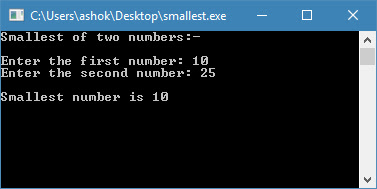This is a simple tutorial for computing smallest of two numbers using the classes and objects in C++. In this tutorial, I have used Dev C++ v5.11 software for compiling the C++ program.Classes and Objects in C++In C++ language, Object Oriented Programming (OOP) is a concept introducted to classify or organize data into objects. A class is a user defined type or data structure declared with keyword class that has data and functions (also called methods) as its members whose access is governed by the three access specifiers private, protected or public (by default access to members of a class is private). The private members are not accessible outside the class; they can be accessed only through methods of the class. The public members form an interface to the class and are accessible outside the class. Instances of a class data type are known as objects and can contain member variables, constants, member functions, and overloaded operators defined by the programmer.Source Code// Smalle…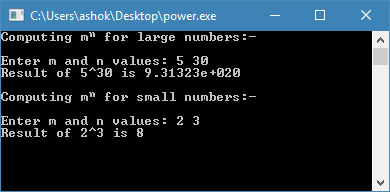add (int a, int b, int c)
i++; // Calling of a function "void operator++()" which assigns x += 5;
i.Print(); // Output is 10Source Code// Computing m^n using function overloading. #include <iostream> #include <conio.h> using namespace std; long double power (double m, int n) { long double x = 1; for (int i = 0; i < n; i++) { x *= m; } return x; } long powe…

### Addition, Subtraction, Multiplication, and Transpose of Matrices using Perl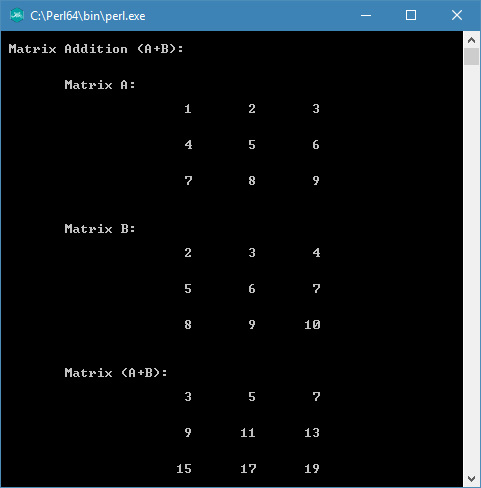This is a Perl programming tutorial for computing addition, subtraction, multiplication, and the transpose of matrices with custom dimension (row × column). In this tutorial, I have used addition(), subtraction(), multiplication(), and transpose() subroutines to perform matrix manipulation by user's choice. Moreover, the input and output of data processing is done through getmatrix() and display() subroutines. The output of the matrix result is formatted in rows and columns mimic to an array.Matrix ManipulationIn mathematics, a matrix is a rectangular array of numbers/symbols/expressions arranged in rows and columns. The individual items (elements or entries) in an m × n matrix A, often denoted by ai,j, where i and j represents the dimension. The rule for addition (A + B) or subtraction (A − B) of two matrices is that it must have an equal number of rows and columns. However, the rule for matrix multiplication (A × B) is that two matrices can be multiplied only when the number of …

### Finding Roots of Quadratic Equation using C++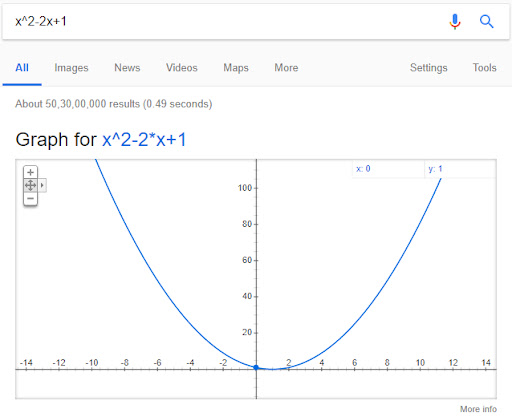This is a simple programming tutorial for finding roots (x1 and x2) of the quadratic equation (ax2 + bx + c = 0) using C++. In this tutorial, I have used Dev C++ v5.11 software for compiling the C++ program.About Quadratic EquationIn algebra, a quadratic equation (quadratussquare → 2) is an equation having the form ax2 + bx + c = 0, where x represents an unknown number (aka variable), and a, b, and c represent known numbers (aka constants).The formula for solving roots of the quadratic equation is $x=\frac{-b\pm \sqrt{{{b}^{2}}-4ac}}{2a}$.
The term b2 - 4ac is known as the determinant (D) of the quadratic equation, which determines the type of the root. Roots of the quadratic equation are broadly categorized into three categories as shown below:DiscriminantFormulaRootsb2 - 4ac = D > 0${{x}_{1}}=\frac{-b+\sqrt{{{b}^{2}}-4ac}}{2a}$Real and Unequal${{x}_{2}}=\frac{-b-\sqrt{{{b}^{2}}-4ac}}{2a}$b2 - 4ac = D = 0${{x}_{1}}={{x}_{2}}=\frac{-b}{2a}$Real and Equalb2 - 4ac = D <…

### Swapping by Call by Reference or Pointer using C++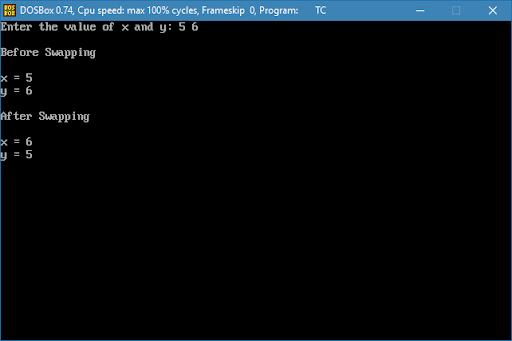This is a simple programming tutorial for swapping values of the two variables by passing parameters to a function using call by reference or call by pointer method in C++. The reference variables are represented by an ampersand symbol (&) before the variable name, and pointer variables are represented by an asterisk symbol (*) before the variable name. In this tutorial, I have used Turbo C++ v3.0 and GNU GCC v7.1.1 software for compiling the C++ program.The values of the two variables can be swapped by initially assigning the value of a variable to a temporary variable in a cyclic order. For instance, a = 1 and b = 2, then t = a, a = b, and b = t.Swapping by Call by ReferenceThe call by reference method of passing arguments to a function copies the reference of an argument into the formal parameter. Inside the function, the reference is used to access the actual argument used in the call. This means that changes made to the parameter affect the passed argument.To pass the value b…

### Temperature Conversion using C++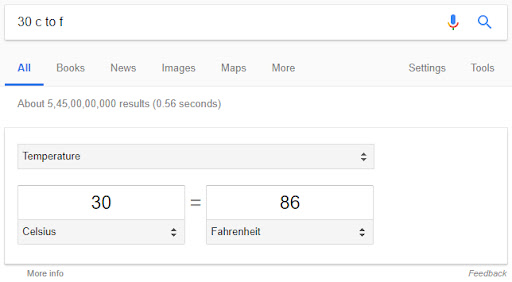This is a simple tutorial for conversion of temperature scales to Celsius or Fahrenheit or Kelvin using C++ program. The same can also easily performed using Google search using the letters c, f, and k. In this tutorial, I have used Turbo C++ v3.0 software for compiling the C++ program.Temperature Conversion and Google SearchThe general temperature conversion formulas and Google search methods such as Fahrenheit to Celsius, Celsius to Fahrenheit, Kelvin to Fahrenheit, Fahrenheit to Kelvin, Celsius to Kelvin, and Kelvin to Celsius are given below.Temperature ConversionFormulaGoogle SearchFahrenheit to Celsius${{T}_{Celsius}}=({{T}_{Fahrenheit}}-32)\times \frac{5}{9}$86 f to cCelsius to Fahrenheit${{T}_{Fahrenheit}}={{T}_{Celsius}}\times \frac{9}{5}+32$30 c to fKelvin to Fahrenheit${{T}_{Fahrenheit}}=\left( {{T}_{Kelvin}}\times \frac{9}{5} \right)-459.67$300 k to fFahrenheit to Kelvin${{T}_{Kelvin}}=({{T}_{Fahrenheit}}+459.67)\times \frac{5}{9}$30 f to kCelsius to Kelvin\[{{T}_{…